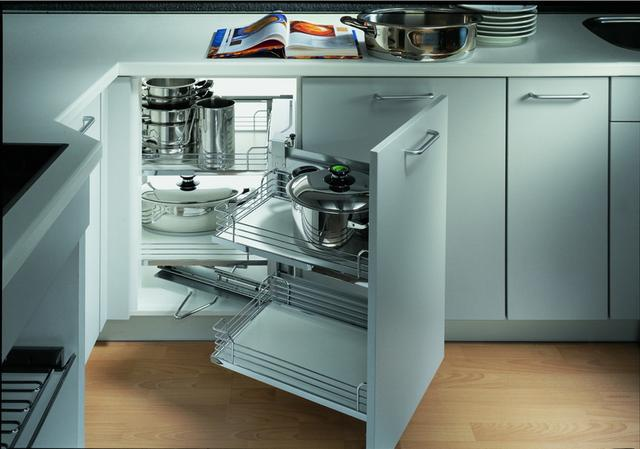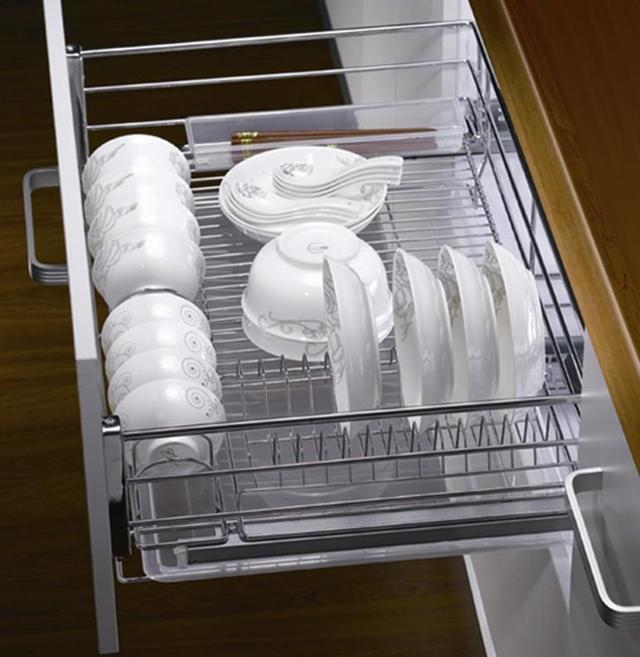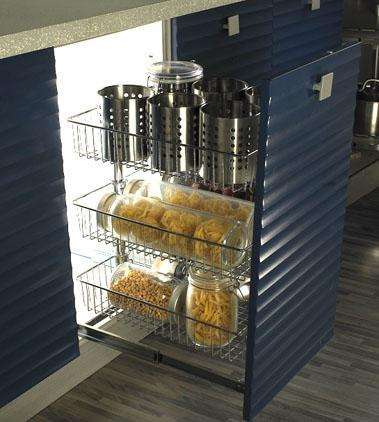|

# 厨房收纳利器 怎能少了橱柜拉篮

厨房中虽然有橱柜的摆放，但是一些琐碎的小件厨房用品像锅碗瓢盆、油盐酱醋等摆放起来十分凌乱。橱柜拉篮的摆放就会让厨房更干净整洁。

拉篮属于五金方面的东西，在橱柜、衣柜里面的比较多，按材质分铁镀铬拉篮、不锈钢、铝合金等，不同的拉篮功能不一样，橱柜里面常用到的功能拉篮是:调料拉篮、碗碟蓝，锅蓝、转角拉篮，怪物拉篮，高深拉篮等。一、橱柜拉篮尺寸大小介绍

由于橱柜拉篮尺寸大小是根据橱柜柜体大小设计的，而我们生活中柜体大小一般有600柜体、700柜体、720柜体、750柜体、800柜体、900柜体五种不同的柜体，对于不同大小的柜体，橱柜拉篮尺寸都有哪些呢?

1、深度42cm的常用橱柜拉篮尺寸(宽*高/cm)：600柜体为52.3*14cm，700柜体为62.3*14cm，720柜体为64.3*14cm，750柜体为66.6*14cm，800柜体为72.3*14cm，900柜体为82.3*14cm。

2、深度45.5cm的常用橱柜拉篮尺寸(宽*高/cm)：600柜体为56.5*20cm，700柜体为66.5*20cm，720柜体为68.5*20cm，750柜体为71.5*20cm，800柜体为76.5*20cm，900柜体为86.5*20cm。二、橱柜拉篮尺寸选择

1、拉篮一般是按橱柜尺寸量身定做，所以提供的橱柜尺寸一定要准确。

2、拉篮的焊点要饱满，无虚焊。

3、拉篮表面要光滑，手感舒适，无毛刺。

4、选择拉篮最重要的一点就是别把镀铬的当成不锈钢的。三、选择拉篮主要注意以下几点。

①拉篮一般是按橱柜尺寸量身定做，所以提供的橱柜尺寸一定要准确。

②拉篮的焊点要饱满，无虚焊。

③拉篮表面要光滑，手感舒适，无毛刺。

④选择拉篮最重要的一点就是别把镀铬的当成不锈钢的。

1、拉篮本身是耐耗品，不易损坏，而其自身的分量越重越增加滑轮承重的压力，减少轨道使用寿命，所以拉篮不是越粗越重越好，但也不能太细，否则容易脱焊，一般主线在8mm就足够了。

2、要做到"一问三看":

"一问"是问材料，因为铁容易生锈，而不锈钢却有很多种，以材料含镍量来区分不锈钢的品质。因为成本和加工工艺难度系数较高的原因，除了广东有一个厂家在生产国标304不锈钢拉篮外，其余工厂均使用201材质生产不锈钢拉篮，不要轻信商家的谎言。广东工厂生产的201不锈钢拉篮质量上有保证，大部分都是201国标料，江浙一带生产的不锈钢拉篮比铁还要便宜，大部分采用废旧金属材料回炉加工而成的不锈钢，品质没有保证。国标不锈钢材料以产地质检部门出示的质检报告单为标准。

"三看":

看外观

首先看外观要整齐而不凌乱，四角皆经过倒角处理，拉篮水平放置在地面上要平衡，底部材料排列要均匀，表面手摸无毛刺无麻点。

看光泽

如果采用电镀处理，应该光亮饱满、外观精美。如果采用电解抛光表面处理，则表面应该呈现不锈钢原有的本色，不得有明显的拉伤刮擦痕迹。

看焊点

焊点不能过大。过大难看，且多为虚焊，不牢固，应略小于相焊的二根材料本身的大小，大公司生产的拉篮焊接点均为碰焊，一般没有焊疤。3、选配置。配置是全套拉篮中最为重要的。

配置一:滑轨，滑轨有一定的承重量，要选材质较厚、顺滑度高、咬合度高、噪音小的滑轨。

配置二:扣件，扣件要选不锈钢材质的。铁片容易生锈且影响门板内侧的美观卫生。

现在大家一定对于橱柜拉篮尺寸应该有了一定的了解了，在以后购买橱柜拉篮时，无论橱柜是订做的，还是常用的整体橱柜尺寸，都要先仔细的量好橱柜的内尺寸，然后才去市场或者橱柜生产厂家咨询，只有这样才能快速的、准确的购买到适当尺寸的橱柜拉篮。

最后，在这里手动打个广告，住好家开通了微信订阅号，大家可以搜索【住好家指南】，我们有专业的设计师可以给大家提供装修建议，只要你提供需求，我们可以给您家免费进行装修方案评估和主材预算评估，并且承诺在24小时完成评估报告，有兴趣的可以添加我们的公众号哦~~

`声明：本文由入驻焦点开放平台的作者撰写，除焦点官方账号外，观点仅代表作者本人，不代表焦点立场错误信息举报电话： 400-099-0099，邮箱：jubao@vip.sohu.com，或点此进行意见反馈，或点此进行举报投诉。`A B C D E F G H J K L M N P Q R S T W X Y Z
A - B - C - D - E
• A
• 鞍山
• 安庆
• 安阳
• 安顺
• 安康
• 澳门
• B
• 北京
• 保定
• 包头
• 巴彦淖尔
• 本溪
• 蚌埠
• 亳州
• 滨州
• 北海
• 百色
• 巴中
• 毕节
• 保山
• 宝鸡
• 白银
• 巴州
• C
• 承德
• 沧州
• 长治
• 赤峰
• 朝阳
• 长春
• 常州
• 滁州
• 池州
• 长沙
• 常德
• 郴州
• 潮州
• 崇左
• 重庆
• 成都
• 楚雄
• 昌都
• 慈溪
• 常熟
• D
• 大同
• 大连
• 丹东
• 大庆
• 东营
• 德州
• 东莞
• 德阳
• 达州
• 大理
• 德宏
• 定西
• 儋州
• 东平
• E
• 鄂尔多斯
• 鄂州
• 恩施
F - G - H - I - J
• F
• 抚顺
• 阜新
• 阜阳
• 福州
• 抚州
• 佛山
• 防城港
• G
• 赣州
• 广州
• 桂林
• 贵港
• 广元
• 广安
• 贵阳
• 固原
• H
• 邯郸
• 衡水
• 呼和浩特
• 呼伦贝尔
• 葫芦岛
• 哈尔滨
• 黑河
• 淮安
• 杭州
• 湖州
• 合肥
• 淮南
• 淮北
• 黄山
• 菏泽
• 鹤壁
• 黄石
• 黄冈
• 衡阳
• 怀化
• 惠州
• 河源
• 贺州
• 河池
• 海口
• 红河
• 汉中
• 海东
• I
• J
• 晋中
• 锦州
• 吉林
• 鸡西
• 佳木斯
• 嘉兴
• 金华
• 景德镇
• 九江
• 吉安
• 济南
• 济宁
• 焦作
• 荆门
• 荆州
• 江门
• 揭阳
• 金昌
• 酒泉
• 嘉峪关
K - L - M - N - P
• K
• 开封
• 昆明
• 昆山
• L
• 廊坊
• 临汾
• 辽阳
• 连云港
• 丽水
• 六安
• 龙岩
• 莱芜
• 临沂
• 聊城
• 洛阳
• 漯河
• 娄底
• 柳州
• 来宾
• 泸州
• 乐山
• 六盘水
• 丽江
• 临沧
• 拉萨
• 林芝
• 兰州
• 陇南
• M
• 牡丹江
• 马鞍山
• 茂名
• 梅州
• 绵阳
• 眉山
• N
• 南京
• 南通
• 宁波
• 南平
• 宁德
• 南昌
• 南阳
• 南宁
• 内江
• 南充
• P
• 盘锦
• 莆田
• 平顶山
• 濮阳
• 攀枝花
• 普洱
• 平凉
Q - R - S - T - W
• Q
• 秦皇岛
• 齐齐哈尔
• 衢州
• 泉州
• 青岛
• 清远
• 钦州
• 黔南
• 曲靖
• 庆阳
• R
• 日照
• 日喀则
• S
• 石家庄
• 沈阳
• 双鸭山
• 绥化
• 上海
• 苏州
• 宿迁
• 绍兴
• 宿州
• 三明
• 上饶
• 三门峡
• 商丘
• 十堰
• 随州
• 邵阳
• 韶关
• 深圳
• 汕头
• 汕尾
• 三亚
• 三沙
• 遂宁
• 山南
• 商洛
• 石嘴山
• T
• 天津
• 唐山
• 太原
• 通辽
• 铁岭
• 泰州
• 台州
• 铜陵
• 泰安
• 铜仁
• 铜川
• 天水
• 天门
• W
• 乌海
• 乌兰察布
• 无锡
• 温州
• 芜湖
• 潍坊
• 威海
• 武汉
• 梧州
• 渭南
• 武威
• 吴忠
• 乌鲁木齐
X - Y - Z
• X
• 邢台
• 徐州
• 宣城
• 厦门
• 新乡
• 许昌
• 信阳
• 襄阳
• 孝感
• 咸宁
• 湘潭
• 湘西
• 西双版纳
• 西安
• 咸阳
• 西宁
• 仙桃
• 西昌
• Y
• 运城
• 营口
• 盐城
• 扬州
• 鹰潭
• 宜春
• 烟台
• 宜昌
• 岳阳
• 益阳
• 永州
• 阳江
• 云浮
• 玉林
• 宜宾
• 雅安
• 玉溪
• 延安
• 榆林
• 银川
• Z
• 张家口
• 镇江
• 舟山
• 漳州
• 淄博
• 枣庄
• 郑州
• 周口
• 驻马店
• 株洲
• 张家界
• 珠海
• 湛江
• 肇庆
• 中山
• 自贡
• 资阳
• 遵义
• 昭通
• 张掖
• 中卫

1室1厅1厨1卫1阳台

1
2
3
4
5

0
1
2

1

1

0
1
2
3报名成功，资料已提交审核A B C D E F G H J K L M N P Q R S T W X Y Z
A - B - C - D - E
• A
• 鞍山
• 安庆
• 安阳
• 安顺
• 安康
• 澳门
• B
• 北京
• 保定
• 包头
• 巴彦淖尔
• 本溪
• 蚌埠
• 亳州
• 滨州
• 北海
• 百色
• 巴中
• 毕节
• 保山
• 宝鸡
• 白银
• 巴州
• C
• 承德
• 沧州
• 长治
• 赤峰
• 朝阳
• 长春
• 常州
• 滁州
• 池州
• 长沙
• 常德
• 郴州
• 潮州
• 崇左
• 重庆
• 成都
• 楚雄
• 昌都
• 慈溪
• 常熟
• D
• 大同
• 大连
• 丹东
• 大庆
• 东营
• 德州
• 东莞
• 德阳
• 达州
• 大理
• 德宏
• 定西
• 儋州
• 东平
• E
• 鄂尔多斯
• 鄂州
• 恩施
F - G - H - I - J
• F
• 抚顺
• 阜新
• 阜阳
• 福州
• 抚州
• 佛山
• 防城港
• G
• 赣州
• 广州
• 桂林
• 贵港
• 广元
• 广安
• 贵阳
• 固原
• H
• 邯郸
• 衡水
• 呼和浩特
• 呼伦贝尔
• 葫芦岛
• 哈尔滨
• 黑河
• 淮安
• 杭州
• 湖州
• 合肥
• 淮南
• 淮北
• 黄山
• 菏泽
• 鹤壁
• 黄石
• 黄冈
• 衡阳
• 怀化
• 惠州
• 河源
• 贺州
• 河池
• 海口
• 红河
• 汉中
• 海东
• I
• J
• 晋中
• 锦州
• 吉林
• 鸡西
• 佳木斯
• 嘉兴
• 金华
• 景德镇
• 九江
• 吉安
• 济南
• 济宁
• 焦作
• 荆门
• 荆州
• 江门
• 揭阳
• 金昌
• 酒泉
• 嘉峪关
K - L - M - N - P
• K
• 开封
• 昆明
• 昆山
• L
• 廊坊
• 临汾
• 辽阳
• 连云港
• 丽水
• 六安
• 龙岩
• 莱芜
• 临沂
• 聊城
• 洛阳
• 漯河
• 娄底
• 柳州
• 来宾
• 泸州
• 乐山
• 六盘水
• 丽江
• 临沧
• 拉萨
• 林芝
• 兰州
• 陇南
• M
• 牡丹江
• 马鞍山
• 茂名
• 梅州
• 绵阳
• 眉山
• N
• 南京
• 南通
• 宁波
• 南平
• 宁德
• 南昌
• 南阳
• 南宁
• 内江
• 南充
• P
• 盘锦
• 莆田
• 平顶山
• 濮阳
• 攀枝花
• 普洱
• 平凉
Q - R - S - T - W
• Q
• 秦皇岛
• 齐齐哈尔
• 衢州
• 泉州
• 青岛
• 清远
• 钦州
• 黔南
• 曲靖
• 庆阳
• R
• 日照
• 日喀则
• S
• 石家庄
• 沈阳
• 双鸭山
• 绥化
• 上海
• 苏州
• 宿迁
• 绍兴
• 宿州
• 三明
• 上饶
• 三门峡
• 商丘
• 十堰
• 随州
• 邵阳
• 韶关
• 深圳
• 汕头
• 汕尾
• 三亚
• 三沙
• 遂宁
• 山南
• 商洛
• 石嘴山
• T
• 天津
• 唐山
• 太原
• 通辽
• 铁岭
• 泰州
• 台州
• 铜陵
• 泰安
• 铜仁
• 铜川
• 天水
• 天门
• W
• 乌海
• 乌兰察布
• 无锡
• 温州
• 芜湖
• 潍坊
• 威海
• 武汉
• 梧州
• 渭南
• 武威
• 吴忠
• 乌鲁木齐
X - Y - Z
• X
• 邢台
• 徐州
• 宣城
• 厦门
• 新乡
• 许昌
• 信阳
• 襄阳
• 孝感
• 咸宁
• 湘潭
• 湘西
• 西双版纳
• 西安
• 咸阳
• 西宁
• 仙桃
• 西昌
• Y
• 运城
• 营口
• 盐城
• 扬州
• 鹰潭
• 宜春
• 烟台
• 宜昌
• 岳阳
• 益阳
• 永州
• 阳江
• 云浮
• 玉林
• 宜宾
• 雅安
• 玉溪
• 延安
• 榆林
• 银川
• Z
• 张家口
• 镇江
• 舟山
• 漳州
• 淄博
• 枣庄
• 郑州
• 周口
• 驻马店
• 株洲
• 张家界
• 珠海
• 湛江
• 肇庆
• 中山
• 自贡
• 资阳
• 遵义
• 昭通
• 张掖
• 中卫• 手机• 分享
• 设计
免费设计
• 计算器
装修计算器
• 入驻
合作入驻
• 联系
联系我们
• 置顶
返回顶部